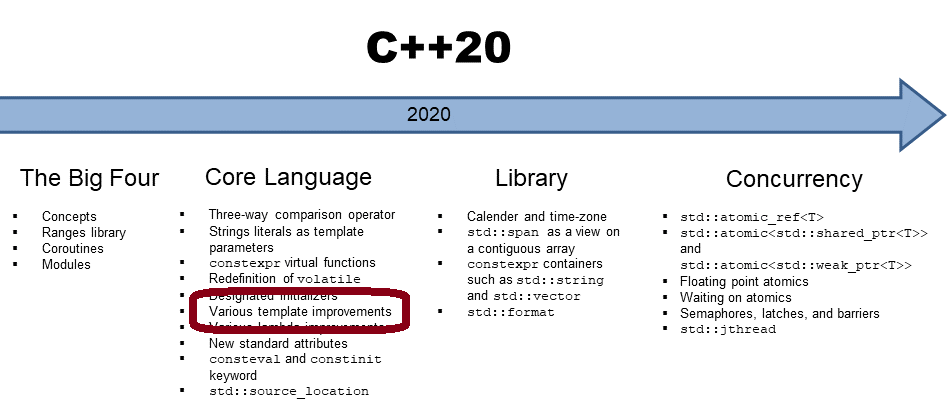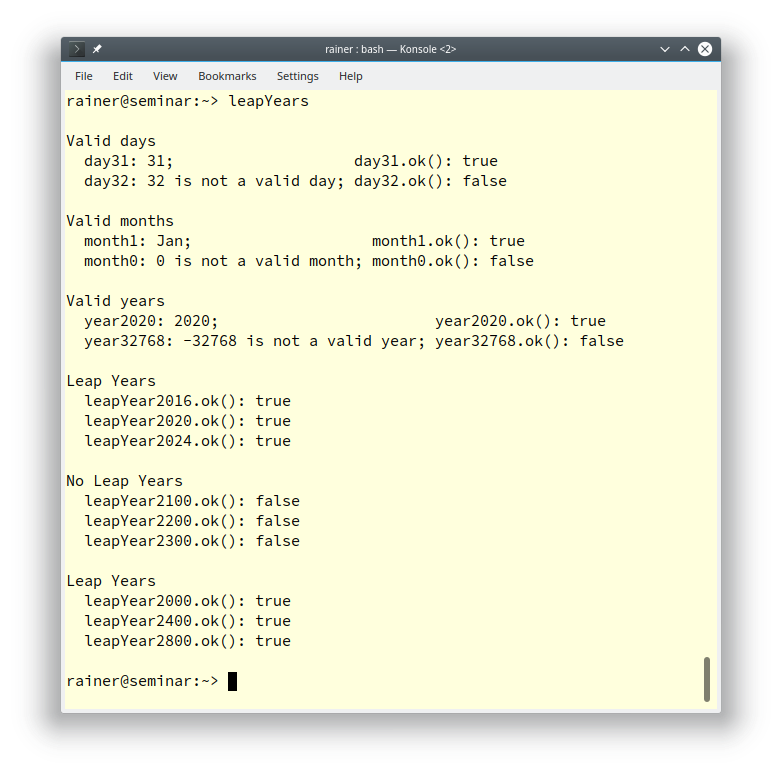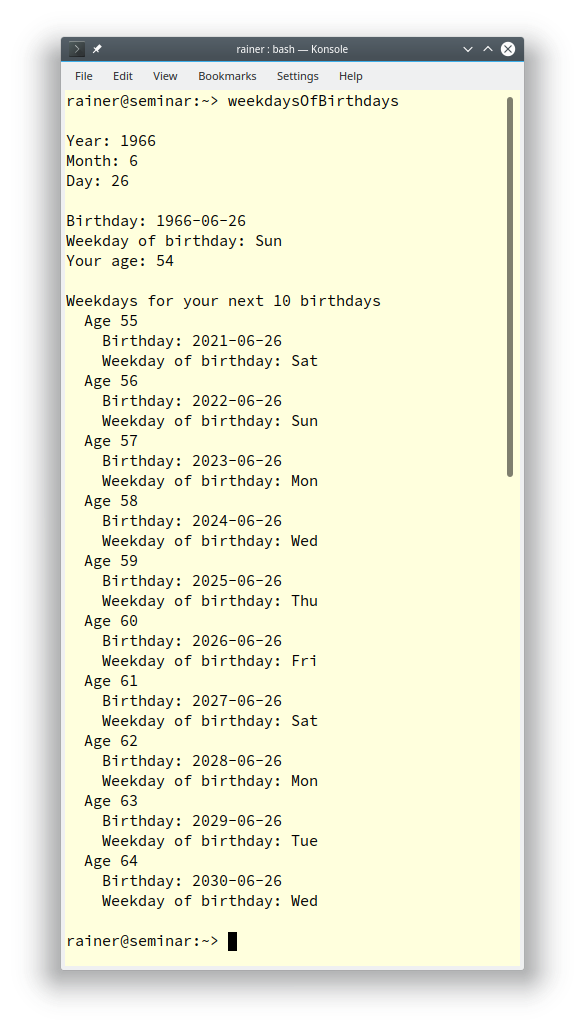# Calendar and Time-Zones in C++20: Handling Calendar Dates

In my last post, “Calendar and Time Zone in C++20: Calendar Dates”, I presented the new calendar-related data types. Today, I go one step further and interact with them.Assume you have a calendar date, such as `year(2100)/2/29. `Your first question may be: Is this date valid?

## Check if a Date is Valid

The various calendar types in C++20 have a function `ok`. This function returns `true` if the date is valid.

```//  leapYear.cpp

#include <iostream>
#include "date.h"

int main() {

std::cout << std::boolalpha << std::endl;

using namespace date;

std::cout << "Valid days" << std::endl;         // (1)
day day31(31);
day day32 = day31 + days(1);
std::cout << "  day31: " << day31 << "; ";
std::cout << "                   day31.ok(): "  << day31.ok() << std::endl;
std::cout << "  day32: " << day32 << "; ";
std::cout << "day32.ok(): "  << day32.ok() << std::endl;

std::cout << std::endl;

std::cout << "Valid months" << std::endl;       // (2)
month month1(1);
month month0(0);
std::cout << "  month1: " << month1 << "; ";
std::cout << "                   month1.ok(): "  << month1.ok() << std::endl;
std::cout << "  month0: " << month0 << "; ";
std::cout << "month0.ok(): "  << month0.ok() << std::endl;

std::cout << std::endl;

std::cout << "Valid years" << std::endl;       // (3)
year year2020(2020);
year year32768(-32768);
std::cout << "  year2020: " << year2020 << "; ";
std::cout << "                       year2020.ok(): "  << year2020.ok() << std::endl;
std::cout << "  year32768: " << year32768 << "; ";
std::cout << "year32768.ok(): "  << year32768.ok() << std::endl;

std::cout << std::endl;

std::cout << "Leap Years"  << std::endl;       // (4)

constexpr auto leapYear2016{year(2016)/2/29};
constexpr auto leapYear2020{year(2020)/2/29};
constexpr auto leapYear2024{year(2024)/2/29};

std::cout << "  leapYear2016.ok(): " << leapYear2016.ok() << std::endl;
std::cout << "  leapYear2020.ok(): " << leapYear2020.ok() << std::endl;
std::cout << "  leapYear2024.ok(): " << leapYear2024.ok() << std::endl;

std::cout << std::endl;

std::cout << "No Leap Years"  << std::endl;   // (5)

constexpr auto leapYear2100{year(2100)/2/29};
constexpr auto leapYear2200{year(2200)/2/29};
constexpr auto leapYear2300{year(2300)/2/29};

std::cout << "  leapYear2100.ok(): " << leapYear2100.ok() << std::endl;
std::cout << "  leapYear2200.ok(): " << leapYear2200.ok() << std::endl;
std::cout << "  leapYear2300.ok(): " << leapYear2300.ok() << std::endl;

std::cout << std::endl;

std::cout << "Leap Years"  << std::endl;      // (6)

constexpr auto leapYear2000{year(2000)/2/29};
constexpr auto leapYear2400{year(2400)/2/29};
constexpr auto leapYear2800{year(2800)/2/29};

std::cout << "  leapYear2000.ok(): " << leapYear2000.ok() << std::endl;
std::cout << "  leapYear2400.ok(): " << leapYear2400.ok() << std::endl;
std::cout << "  leapYear2800.ok(): " << leapYear2800.ok() << std::endl;

std::cout << std::endl;

}
```

##Modernes C++ Mentoring

Be part of my mentoring programs:

• "Fundamentals for C++ Professionals" (open)
• "Design Patterns and Architectural Patterns with C++" (open)
• "C++20: Get the Details" (reopens December 2023)
• Do you want to stay informed about my mentoring programs: Subscribe via E-Mail.

I checked in the program if a given day (line 1), a given month (line 2), or a given year (line 3) is valid. The range of a day is [1, 31], of a month [1, 12], and of a year [ -32767, 32767]. Consequently, the` ok`-call on the corresponding values returns `false`. Two facts are interesting when I output the various values. First, if the value is not valid, the output displays: `"is not a valid day`“; `"is not a valid month`“; `"is not a valid year`“. Second, month values are displayed in string representation.You can apply the `ok`-call on a calendar date. Now it’s pretty easy to check if a specific calendar date is a leap day and, therefore, the corresponding year a leap year. In the worldwide used Gregorian calendar, the following rules apply:

Each year that is exactly divisible by 4 is a leap year.

• Except for years that are exactly divisible by 100. They are no leap years.
• Except for years that are exactly divisible by 400. They are leap years.

Too complicate? The program leapYears.cpp exemplifies this rule.

The extended chrono library makes it quite comfortable to ask for the duration between calendar dates.

## Query Calendar Dates

Without further ado. The following program queries a few calendar dates.

```// queryCalendarDates.cpp

#include "date.h"
#include <iostream>

int main() {

using namespace date;

std::cout << std::endl;

auto now = std::chrono::system_clock::now();              // (1)
std::cout << "The current time is: " << now << " UTC\n";
std::cout << "The current date is: " << floor<days>(now) << std::endl;
std::cout << "The current date is: " << year_month_day{floor<days>(now)} << std::endl;
std::cout << "The current date is: " << year_month_weekday{floor<days>(now)} << std::endl;

std::cout << std::endl;

auto currentDate = year_month_day(floor<days>(now));       // (2)
auto currentYear = currentDate.year();
std::cout << "The current year is " << currentYear << '\n';
auto currentMonth = currentDate.month();
std::cout << "The current month is " << currentMonth << '\n';
auto currentDay = currentDate.day();
std::cout << "The current day is " << currentDay << '\n';

std::cout << std::endl;
// (3)
auto hAfter = floor<std::chrono::hours>(now) - sys_days(January/1/currentYear);
std::cout << "It has been " << hAfter << " since New Year!\n";
auto nextYear = currentDate.year() + years(1);             // (4)
auto nextNewYear = sys_days(January/1/nextYear);
auto hBefore =  sys_days(January/1/nextYear) - floor<std::chrono::hours>(now);
std::cout << "It is " << hBefore << " before New Year!\n";

std::cout << std::endl;
// (5)
std::cout << "It has been " << floor<days>(hAfter) << " since New Year!\n";
std::cout << "It is " << floor<days>(hBefore) << " before New Year!\n";

std::cout << std::endl;

}
```

With the C++20 extension, you can directly display a time point, such as now (line 1). `std::chrono::floor` allows it to convert the time point to a day `std::chrono::sys_days`. This value can be used to initialize the calendar type` std::chrono::year_month_day`. Finally, when I put the value into a `std::chrono::year_month_weekday` calendar type, I get the answer that this day is the 3rd Tuesday in October.

Of course, I can also ask for a calendar date for its components, such as the current year, month, or day (line 2).

Line (3) is the most interesting one. When subtracting from the current date in the hour’s resolution first January of the current year, I get the hour since the new year. On the contrary: When I subtract from the first January of the following year (line 4) the current date in hours resolution, I get the hours to the new year. Maybe you don’t like the hour’s resolution. Line 5 displays the values in days resolution.I want to know the weekdays of my birthdays.

## `Query Weekdays`

Thanks to the extended chrono library, getting the weekday of a given calendar date is pretty easy.

```// weekdaysOfBirthdays.cpp

#include <cstdlib>
#include <iostream>
#include "date.h"

int main() {

std::cout << std::endl;

using namespace date;

int y;
int m;
int d;

std::cout << "Year: ";                          // (1)
std::cin >> y;
std::cout << "Month: ";
std::cin >> m;
std::cout << "Day: ";
std::cin >> d;

std::cout << std::endl;

auto birthday = year(y)/month(m)/day(d);       // (2)

if (not birthday.ok()) {                       // (3)
std::cout << birthday << std::endl;
std::exit(EXIT_FAILURE);
}

std::cout << "Birthday: " << birthday << std::endl;
auto birthdayWeekday = year_month_weekday(birthday);    // (4)
std::cout << "Weekday of birthday: " << birthdayWeekday.weekday() << std::endl;

auto currentDate = year_month_day(floor<days>(std::chrono::system_clock::now()));
auto currentYear = currentDate.year();

auto age = (int)currentDate.year() - (int)birthday.year();  // (5)
std::cout << "Your age: " << age << std::endl;

std::cout << std::endl;

std::cout << "Weekdays for your next 10 birthdays" << std::endl;  // (6)

for (int i = 1, newYear = (int)currentYear; i <= 10;  ++i ) {
std::cout << "  Age " <<  ++age << std::endl;
auto newBirthday = year(++newYear)/month(m)/day(d);
std::cout << "    Birthday: " << newBirthday << std::endl;
std::cout << "    Weekday of birthday: "
<< year_month_weekday(newBirthday).weekday() << std::endl;
}

std::cout << std::endl;

}
```

First, the program asks you for the year, month, and day of your birthday (line 1). Based on the input, a calendar date is created (line 2) and checked for validity (line 3). Now I display the weekday of your birthday. I use, therefore, the calendar date to fill the calendar type `std::chrono::year_month_weekday` (line 4). To get the `int` representation of the calendar-type year, I have to convert it to `int` (line 5). Now I can display your age. Finally, the for-loop displays the following information for each of your next ten birthdays (line 6): your age, the calendar date, and the weekday. I only have to increment the `age` and `newYear` variable.

Here is a run of the program with my birthday.## What’s next?

A critical component in my posts to the extended Chrono library is still missing: time zones.

Thanks a lot to my Patreon Supporters: Matt Braun, Roman Postanciuc, Tobias Zindl, G Prvulovic, Reinhold Dröge, Abernitzke, Frank Grimm, Sakib, Broeserl, António Pina, Sergey Agafyin, Андрей Бурмистров, Jake, GS, Lawton Shoemake, Jozo Leko, John Breland, Venkat Nandam, Jose Francisco, Douglas Tinkham, Kuchlong Kuchlong, Robert Blanch, Truels Wissneth, Kris Kafka, Mario Luoni, Friedrich Huber, lennonli, Pramod Tikare Muralidhara, Peter Ware, Daniel Hufschläger, Alessandro Pezzato, Bob Perry, Satish Vangipuram, Andi Ireland, Richard Ohnemus, Michael Dunsky, Leo Goodstadt, John Wiederhirn, Yacob Cohen-Arazi, Florian Tischler, Robin Furness, Michael Young, Holger Detering, Bernd Mühlhaus, Matthieu Bolt, Stephen Kelley, Kyle Dean, Tusar Palauri, Dmitry Farberov, Juan Dent, George Liao, Daniel Ceperley, Jon T Hess, Stephen Totten, Wolfgang Fütterer, Matthias Grün, Phillip Diekmann, Ben Atakora, Ann Shatoff, Rob North, Bhavith C Achar, and Marco Parri Empoli.

Thanks, in particular, to Jon Hess, Lakshman, Christian Wittenhorst, Sherhy Pyton, Dendi Suhubdy, Sudhakar Belagurusamy, Richard Sargeant, Rusty Fleming, John Nebel, Mipko, Alicja Kaminska, Slavko Radman, and David Poole.

 My special thanks to EmbarcaderoMy special thanks to PVS-StudioMy special thanks to Tipi.buildMy special thanks to Take Up Code## Seminars

I’m happy to give online seminars or face-to-face seminars worldwide. Please call me if you have any questions.

### Standard Seminars (English/German)

Here is a compilation of my standard seminars. These seminars are only meant to give you a first orientation.

• C++ – The Core Language
• C++ – The Standard Library
• C++ – Compact
• C++11 and C++14
• Concurrency with Modern C++
• Design Pattern and Architectural Pattern with C++
• Embedded Programming with Modern C++
• Generic Programming (Templates) with C++

#### New

• Clean Code with Modern C++
• C++20

### Contact Me

Modernes C++ Mentoring,Tags:
0 replies